Electromagnetic field analysis software|ELF Corporation

The ELF series is currently used by many companies, research institutes, and universities.
This software calculates electromagnetic fields using the integral equation method (IEM).
In many cases, even when it is difficult to analyze by FEM (Finite Element Method) or BEM (Boundary Element Method), it can be easily analyzed.

In general, IEMs have the potential to analyze electromagnetic fields with high speed and accuracy.
Although orthodox IEMs have a problem with stability of the solution (example of orthodox IEM ≫)
We have clarified the cause of this problem and made our own improvements.
As a result, we were able to establish a calculation method that can obtain a solution with high speed and high accuracy.

## Features

#### Spatial magnetic field and spatial electric field with high accuracy without spatial mesh ∨

No spatial mesh is required because the integral form of Maxwell's equations is used.
The mesh is easy to create.
Electric and magnetic fields in space are highly accurate because the analytical expressions from the source are used.
Calculation of the trajectory of charged particles in space is highly accurate.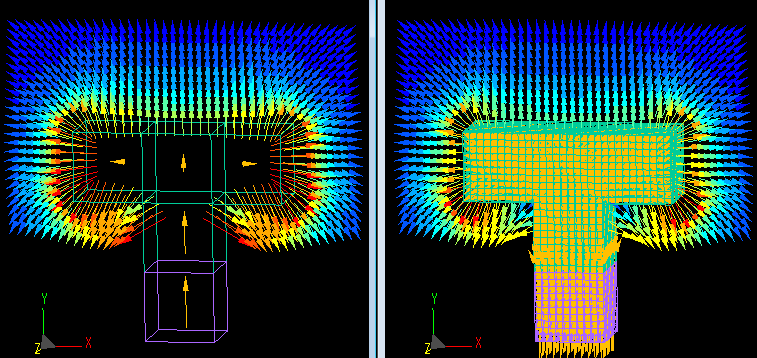#### High-speed and high-accuracy calculation with a small number of elements ∨

With the same number of elements, the IEM can be calculated with much higher accuracy than the FEM.
As a result, it is faster than FEM for the same accuracy.
There are examples of calculations that are 2000 times faster than FEM.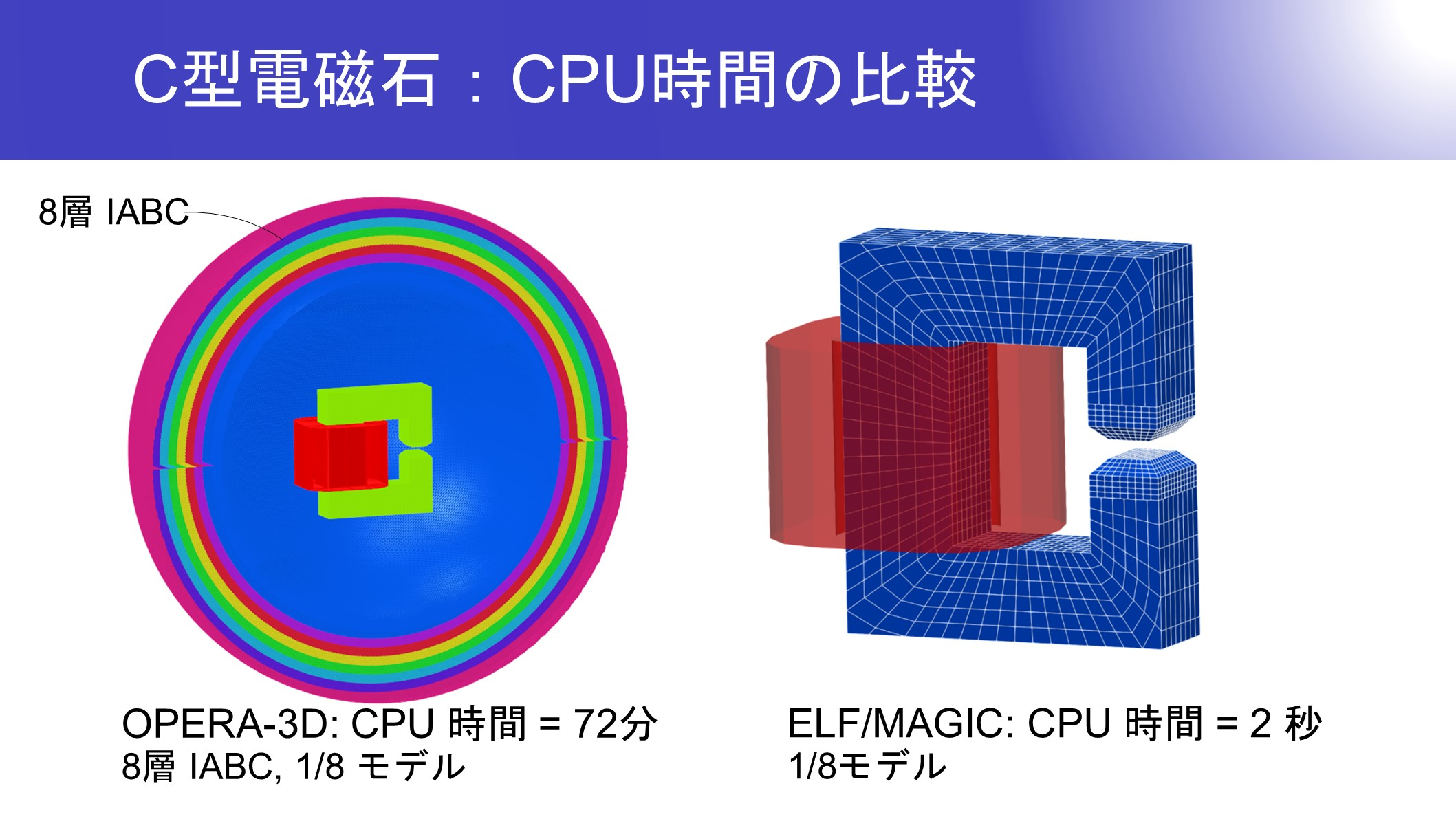#### Motion of bodies ∨

When multiple bodies are in motion, there is no need to remesh because no mesh is needed in the space between the bodies.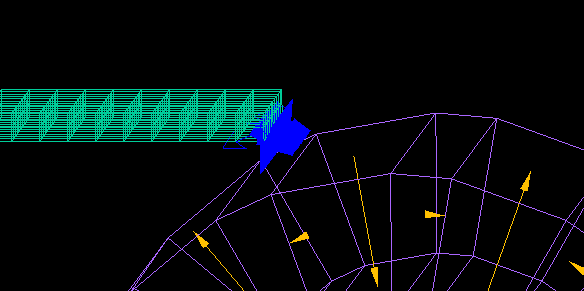#### Force acting on bodies in close proximity ∨

Both attractive and repulsive forces can be accurately calculated by analytical formulas until they are very close together.
This is the calculation of the force acting when two magnets of 1 cm on each side are brought closer together from 1 cm to 0.1 μ.
Both the attractive and repulsive forces converge to the same value even when the gap is narrow.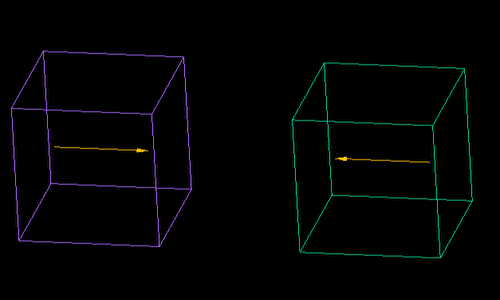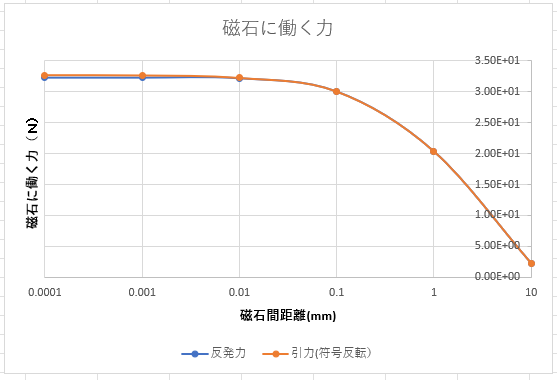Even when charged particles are in close proximity to magnets or electrodes, the analytical formula ensures that accuracy is not decreased.

#### Mixture of parts with different scales ∨

If the two parts are separated, the continuity of the mesh is not a question, so the mesh size can be extremely large or small.

#### Very thin magnetic materials ∨

If there are very thin magnetic materials in the space, it is easy because no space mesh is needed.

#### Handling of magnets ∨

Magnets can be analyzed with extremely coarse meshes because there are little changes in the internal magnetization even when an external magnetic field acts on them.

Analysis of magnetization is also possible.

#### Handling of coils ∨

The division of coil elements is easy because it can be done almost independently of the division of air and magnetic elements.
Long straight coil does not need to be divided.

#### Type of analysis ∨

3D Analysis, 2D Analysis, Axisymmetric Analysis
static magnetic field analysis, dynamic magnetic field analysis, eddy current analysis, transient analysis, sinusoidal analysis, static electric field analysis, charged particle trajectory analysis

#### Objects that can be handled ∨

Magnetic materials, magnets, coils, conductors, magnetic conductors, electrodes, dielectrics

#### Physical quantity that can be calculated ∨

Magnetic and electric fields, magnetic and electric flux density
eddy currents, charge density, forces, motion
Induced currents, Joule heat, interlinkage flux, inductance, charged particle trajectory

#### Applications ∨

Electron microscopes, sensors, magnet products, magnetic shields, motors, linear motors, relays, plungers,transformer
Electron holography, sputtering, MEMS, magnetic heads, flat cathode ray tubes
electron guns, accelerators, capacitors

## We exhibited at Compumag 2023.

Dates: Monday, May 22 - Friday, May 26, 2023
Venue: Kyoto International Conference Center
https://www.compumag2023.com/

## Usage

#### Operation Guide ∨

How to use each tool and how to create data.
Operation Guide ≫

Price List ≫